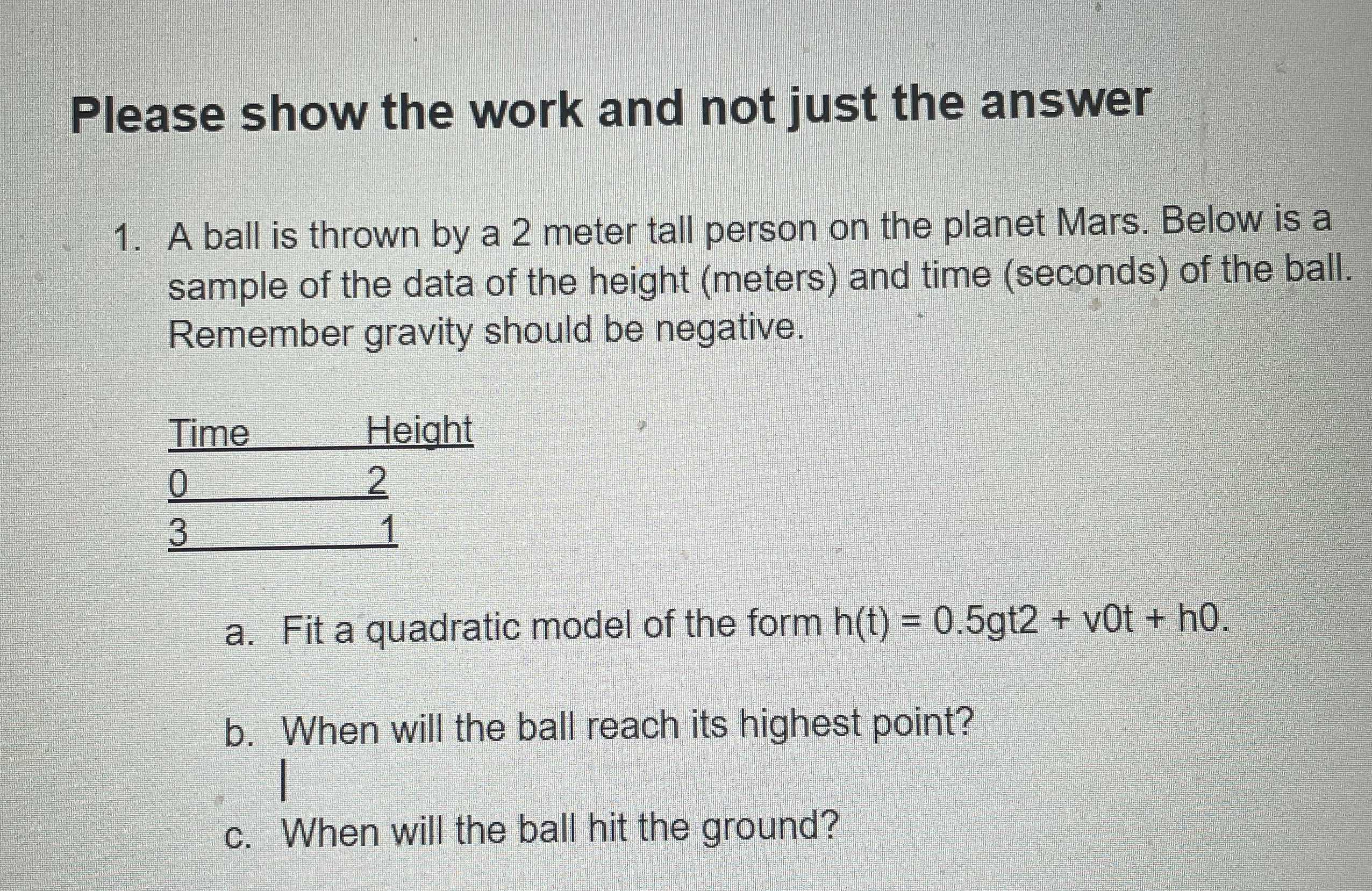### ¿Todavía tienes preguntas de matemáticas?

Pregunte a nuestros tutores expertos
Algebra
PreguntaA ball is thrown by a $$2$$ meter tall person on the planet Mars. Below is a sample of the data of the height (meters) and time (seconds) of the ball. Remember gravity should be negative.

a. Fit a quadratic model of the form $$h ( t ) = 0.5 gt 2 + v 0 t + h 0$$ .

b. When will the ball reach its highest point?

$$h(t)= 0.5gt^2- 6t+ 2$$
$$x= \frac{6}{g}$$